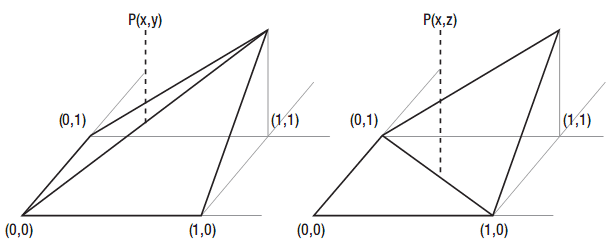# 5.9 使用双线性插值计算地形的精确高度

## 工作原理

### 线性插值

0.7* (maxY－min Y)＋minY=0.7*(20－10)＋10=0.7*10＋10=17

14→(14－minX)/(maxX－minX) =(14－13)/(16－13)=0.33

0.33* (maxY－minY)＋minY=0.33*(46－35)＋35=0.33*11＋35=3.67＋35=38.67

### 获取对应值

int xLower = (int)xCoord;
int xHigher = xLower + 1;
float xRelative = (xCoord - xLower) / ((float)xHigher - (float)xLower);
int zLower = (int)zCoord;
int zHigher = zLower + 1;
float zRelative = (zCoord - zLower) / ((float)zHigher- (float)zLower);

### 获取minY和maxY的值

float heightLxLz = heightData[xLower, zLower];
float heightLxHz = heightData[xLower, zHigher];
float heightHxLz = heightData[xHigher, zLower];
float heightHxHz = heightData[xHigher, zHigher]; 

LxHz表示“低X坐标，高Z坐标” 决定(X,Z)。bool pointAboveLowerTriangle = (xRelative + zRelative < 1);

### 获取精确高度

finalHeight = heightLxLz;
finalHeight += zRelative * (heightLxHz - heightLxLz);
finalHeight += xRelative * (heightHxLz - heightLxLz); 

finalHeight = heightHxHz;
finalHeight += (1.0f - zDifference) *(heightHxLz - heightHxHz);
finalHeight += (1.0f - xDifference) * (heightLxHz - heightHxHz); 

## 代码

public float GetExactHeightAt(float xCoord, float zCoord)
{
bool invalid = xCoord < 0;
invalid |= zCoord < 0;
invalid |= xCoord > heightData.GetLength(0) - 1;
invalid |= zCoord > heightData.GetLength(1) - 1;

if (invalid)
return 10;

int xLower = (int)xCoord;
int xHigher = xLower + 1;
float xRelative = (xCoord - xLower) / ((float)xHigher - (float)xLower);
int zLower = (int)zCoord;
int zHigher = zLower + 1;
float zRelative = (zCoord - zLower) / ((float)zHigher - (float)zLower);
float heightLxLz = heightData[xLower, zLower];
float heightLxHz = heightData[xLower, zHigher];
float heightHxLz = heightData[xHigher, zLower];
float heightHxHz = heightData[xHigher, zHigher];

bool pointAboveLowerTriangle = (xRelative + zRelative < 1);
float finalHeight;
if (pointAboveLowerTriangle )
{
finalHeight = heightLxLz;
finalHeight += zRelative * (heightLxHz - heightLxLz);
finalHeight += xRelative * (heightHxLz - heightLxLz);
}
else
{
finalHeight = heightHxHz;
finalHeight += (1.0f - zRelative) * (heightHxLz - heightHxHz);
finalHeight += (1.0f - xRelative) * (heightLxHz - heightHxHz);
}
return finalHeight; } 

2006 - 2023，推荐分辨率1024*768以上，推荐浏览器Chrome、Edge等现代浏览器，截止2021年12月5日的访问次数：1872万9823 站长邮箱